Mathematical and Physical Journal
for High Schools
Issued by the MATFUND Foundation
 Already signed up? New to KöMaL?

# KöMaL Problems in Physics, April 2011

Show/hide problems of signs:## Problems with sign 'M'

Deadline expired on May 10, 2011.

M. 314. Measure how the temperature of a pot of water placed to a gas stove changes as a function of the elapsed time,

a) If there is a lid on the pot;

b) if there is no lid on the pot.

(6 pont)

statistics## Problems with sign 'P'

Deadline expired on May 10, 2011.

P. 4337. According to the Atlas of explorations and inventions'' the the viking ships were 24 m long, 5.2 m wide and they emerged into the water to a depth of 1.8 m. Estimate the carrying capacity of the ships if the depth to which they emerged increased by 10% when they were fully loaded.

(3 pont)

solution (in Hungarian), statistics

P. 4338. Determine how the centre of weight (mass) of a trapezium shaped sheet can be constructed.

(4 pont)

solution (in Hungarian), statistics

P. 4339. Similar rubber bungs are stick together as shown in the figure. The bodies are placed to a pair of rails, which encloses a small angle with the horizontal. The distance between the rails is equal to the height of the original bungs. We experience with a surprise that one of the bodies always reach the bottom of the slope, and the other always gets derailed. Which one? (The bodies roll without sliding.)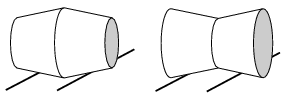(4 pont)

solution (in Hungarian), statistics

P. 4340. A small ball of mass m=0.4 kg is hanging on a thread of length=1 m, and touches another ball of mass m and of the same radius. This second ball is on a horizontal plane and is attached to one end of a horizontal spring of spring constant D=10 N/m, as shown in the figure. Initially the spring is unstretched and its other end is fixed. The simple pendulum is deflected at an angle of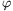=20o, and it is released without any initial speed.

a) At what speed do the two balls collide? What is the percentage error that is made if the speed of the ball is calculated from the formula of the period of the pendulum.

b) How much time elapses until the pendulum gets back again to the position where its displacement is the greatest, having collided with the other ball totally elastically? (Friction is negligible.)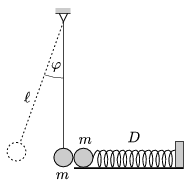(5 pont)

solution (in Hungarian), statistics

P. 4341. A long slope of angle of elevationis made of solid rubber cylinders which can easily revolve about their fixed axles. The rollers, which have mass of m and radius R, are close to each other, but they do not touch each other. To what maximum speed can a crate of mass M be accelerated? (The coefficient of friction between the crate and the rollers is big enough.)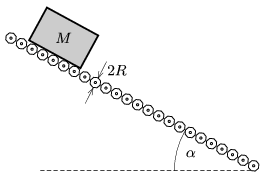(5 pont)

solution (in Hungarian), statistics

P. 4342. There are three vases on the table, none of them is taller than 40 cm. The hole in all of them is a vertical cylinder. The first vase can be filled with 2 dl water, the second one with 3 dl, and the third with more than 6 dl water. The area of the cross section of the first vase is 10 cm2, the area of the cross section of the other two vases is 20 cm2. There is the same amount of water in each vase. It can be observed that if a specific tuning fork is placed just above the vases, its sound is getting louder. The width of the bottom of each vase is 1 cm, the speed of sound in air is 340 m/s, and the frequency of the tuning fork is less than 1 kHz.

a) What is the amount of water in each vase?

b) What is the frequency of the tuning fork?

c) What is the height of the third vase?

(5 pont)

solution (in Hungarian), statistics

P. 4343. The three cylinders shown in the figure have the same cross section. In the cylinders there is the same type of ideal gas, and all the cylinders are closed with easily movable pistons of the same mass. The pistons are rigidly attached to each other. Initially the three samples of gas have the same density, the same temperature of t=27 oC, and the same height of=20 cm.

How much do the pistons rise if the temperature of the gas in the middle cylinder is increased to t'=117 oC, while the temperature of the gas in the two bordering cylinders is kept constant?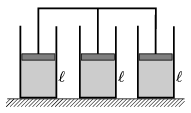(4 pont)

solution (in Hungarian), statistics

P. 4344. A capacitor and a resistor of big resistance, through which the capacitor is charged slowly, are connected in series to a battery of electromotive force 4.5 V. The voltage across the capacitor is 3 V one minute after the closing the circuit. What will the voltage across the capacitor be

a) after 2 minutes;

b) after 3 minutes?

(4 pont)

solution (in Hungarian), statistics

P. 4345. A converging lens of optical power 2 dioptre and of diameter 8 cm is stick to one end of a 30 cm long cardboard tube, and a concave lens of optical power -5 dioptre and of the same diameter is stick to the other end of the tube. The tube is held such that it points towards the Sun with the converging lens. A screen is placed first at a distance of 10 cm from the diverging lens, and then at a distance of 20 cm.

What can be seen on the screen?

(4 pont)

solution (in Hungarian), statistics

P. 4346. A hundred years ago in 1911 Rutherford wrote in his article, that the speed of anparticle, which is moving towards the centre of a gold atom, decreases to zero at that distance, measured from the centre of the gold atom, where its electric potential energy is equal to its initial kinetic energy. The electric potential energy is partly due to the positive charge at the centre of the atom, and partly due to the negative charge which is uniformly distributed in the atom. The radius of the gold nucleus is 10-10 m.

What is this particular distance, if the speed of theparticle is 2.107 m/s?

(5 pont)

solution (in Hungarian), statistics

P. 4347. What is the energy of the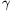photon which can create a positron when it collides with an electron at rest? The rest mass of the electron (and the positron) is 510 keV.

(6 pont)

solution (in Hungarian), statistics